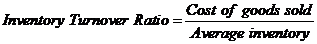# Use a spreadsheet and the following excerpts from Hileah Company’s financial information to build a template that automatically calculates (A) inventory turnover and (B) number of days’ sales in inventory, for the year 2018.FindFindarrow_forward

### Principles of Accounting Volume 1

19th Edition
OpenStax
Publisher: OpenStax College
ISBN: 9781947172685

#### Solutions

Chapter
SectionFindFindarrow_forward

### Principles of Accounting Volume 1

19th Edition
OpenStax
Publisher: OpenStax College
ISBN: 9781947172685
Chapter 10, Problem 6TP
Textbook Problem
1 views

## Use a spreadsheet and the following excerpts from Hileah Company’s financial information to build a template that automatically calculates (A) inventory turnover and (B) number of days’ sales in inventory, for the year 2018.To determine

Concept introduction:

Inventory Turnover Ratio:

Inventory Turnover Ratio measures the efficiency of the company in converting its inventory into sales. It is calculated by dividing the Cost of goods sold by Average inventory. The formula of the Inventory Turnover Ratio is as follows:

Inventory Turnover Ratio=Cost of goods soldAverage inventory

Note: Average inventory is calculated with the help of following formula:

Average inventory=(Beginning inventory + Ending inventory)2

Day’s sales in inventory:

Days sales in inventory represent the number of days the inventory waits for the sale. It is calculated using the following formula:

Day Sales in Inventory =  Inventory *365Cost of Goods Sold

Requirement-1:

To calculate:

The Inventory Turnover Ratio.

### Explanation of Solution

The Inventory Turnover Ratio and is calculated as follows:

 Year 2018 Cost of Goods Sold (A) \$ 177,000 Beginning Inventory (B)
To determine

Concept introduction:

Inventory Turnover Ratio:

Inventory Turnover Ratio measures the efficiency of the company in converting its inventory into sales. It is calculated by dividing the Cost of goods sold by Average inventory. The formula of the Inventory Turnover Ratio is as follows:

Inventory Turnover Ratio=Cost of goods soldAverage inventory

Note: Average inventory is calculated with the help of following formula:

Average inventory=(Beginning inventory + Ending inventory)2

Day’s sales in inventory:

Days sales in inventory represent the number of days the inventory waits for the sale. It is calculated using the following formula:

Day Sales in Inventory =  Inventory *365Cost of Goods Sold

Requirement-2:

To calculate:

The Days sales in inventory for the year 2018.

### Still sussing out bartleby?

Check out a sample textbook solution.

See a sample solution

#### The Solution to Your Study Problems

Bartleby provides explanations to thousands of textbook problems written by our experts, many with advanced degrees!

Get Started